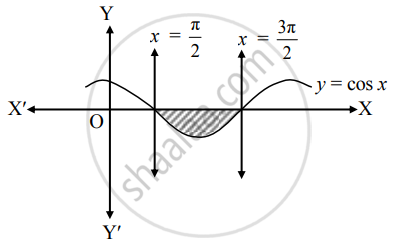# Find the area enclosed between y = cos x and X-axis between the lines x = π2 and x ≤ 3π2 - Mathematics and Statistics

Sum

Find the area enclosed between y = cos x and X-axis between the lines x = pi/2 and x ≤ (3pi)/2

#### Solution

Let A be the required area.

Consider the equation y = cos x.∴ A = int_(pi/2)^((3pi)/2) y  "d"x

= int_(pi/2)^((3pi)/2) cos x  "d"x

= [sin x]_(pi/2)^((3pi)/2)

= sin  (3pi)/2 - sin  pi/2

= –1 – 1

= – 2

But, area cannot be negative.

∴ A = 2 sq.units

Concept: Area Between Two Curves
Is there an error in this question or solution?